# Areas Of Parallelograms And Triangles Class 9 Notes: Chapter 9

Areas Of Parallelograms and Triangles Class 9 Notes are provided here so that students can study effectively and complete the entire revision of chapter 9 and the topics before the exams. The notes provided here contain clear and concise explanations of the concepts and will help students perform better in the exams. Some of the important topics covered in chapter 9 notes include;

• Introduction to areas of parallelograms and triangles.
• Figures on the same base and between the same parallels.
• Parallelograms on the same base and between the same parallels.
• Triangles on the same base and between the same parallels.
• Examples.
• Important questions.

## Introduction To Areas Of Parallelograms And Triangles

If P and Q are congruent figures, then area(P) = area(Q). Two congruent figures will always have equal areas. However, it is not compulsory that two figures equal in an area will be congruent. Area of parallelograms lying on same or equal base and parallel lines are equal. If a triangle and a parallelogram are lying on the same base and same parallel lines, then, the area of the triangle is half the area of the parallelogram.

Area of triangles lying on the same base and parallel lines are equal. A median divides a triangle into two triangles equal in area. Now, let’s prove that a median divides a triangle into 2 triangles having equal areas. Let us consider a triangle ABC with AD as one of its medians. We need to prove that area of triangle ABD = area of triangle ACD.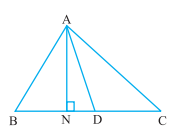Let us draw AN perpendicular to BC. Now area of triangle ABD = ½ × base × altitude = ½ BD × AN = ½ CD × AN [Since, BD and CD are equal]

= ½ × base × altitude (of ∆ ACD) = area of triangle ACD

### Figures on the Same Base and Between the Same Parallels

Theorem: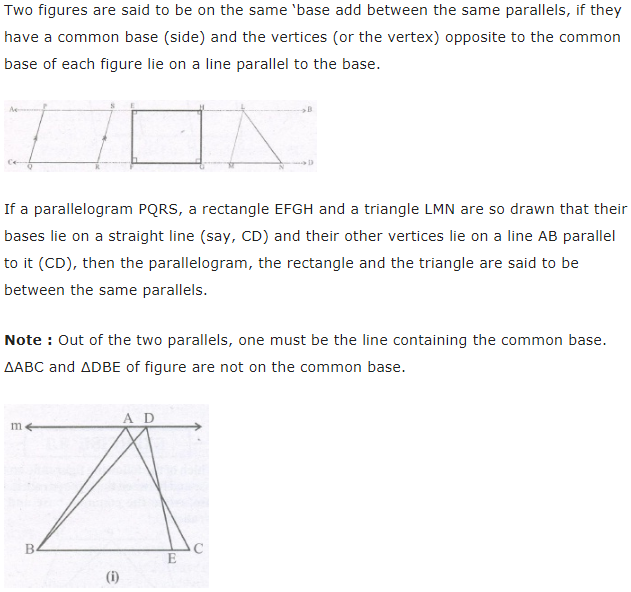### Parallelograms on the same Base and Between the same Parallels

Theorem: Parallelograms on the same base and between the same parallel sides are equal in area.

Let’s take an instance where two parallelograms exist ABCD and ABEF on the same base DC and between the same parallel line AB and FC. Here we will try to prove that area (ABCD) = area (ABEF).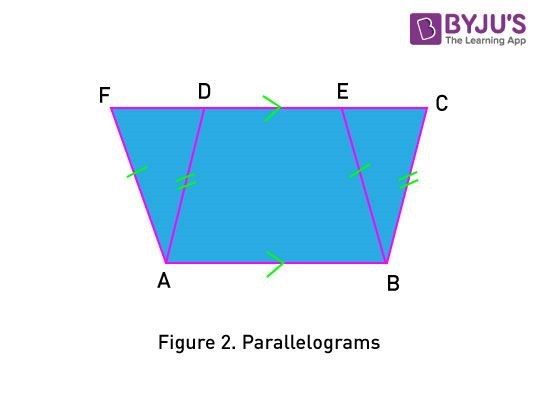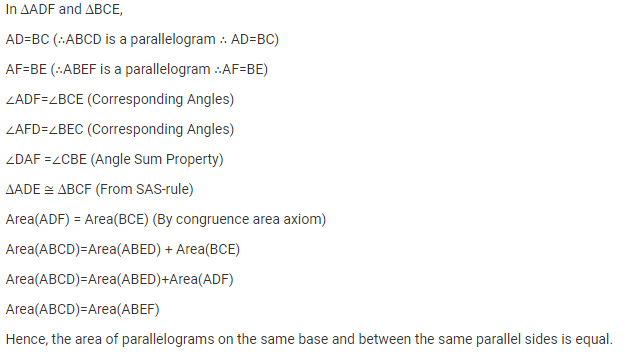### Triangles on the same Base and between the same Parallels

Theorem: Two triangles on the same base (or equal bases) and between the same parallel sides are equal in area.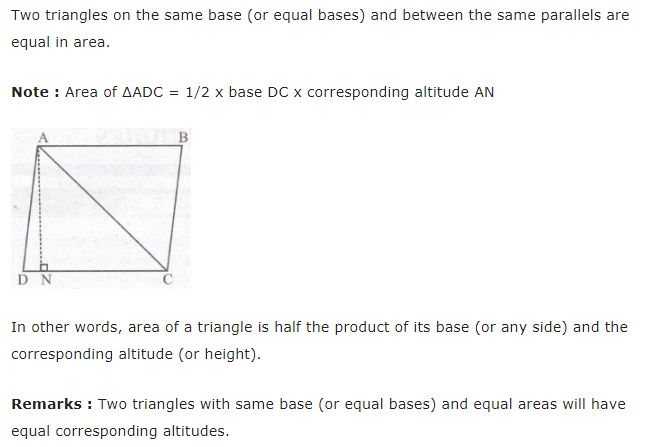#### Examples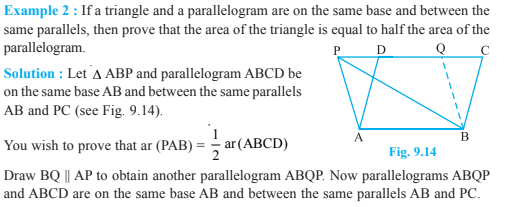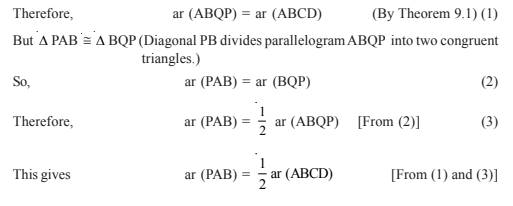##### Important Questions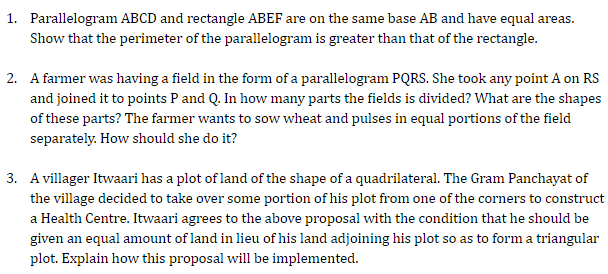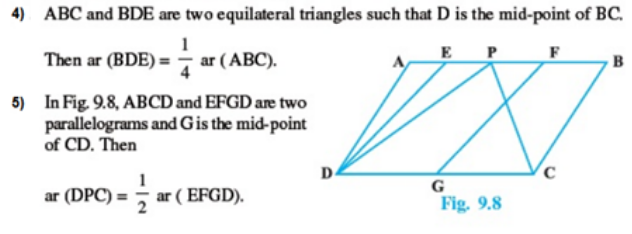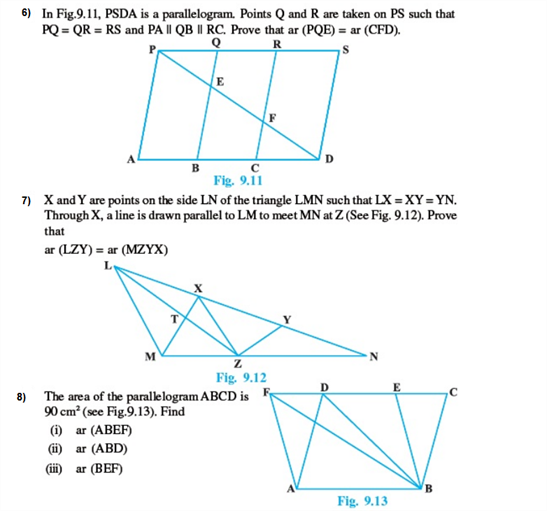Also Access NCERT Exemplar for class 9 Maths Chapter 9